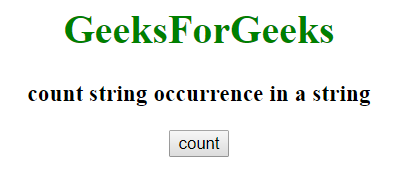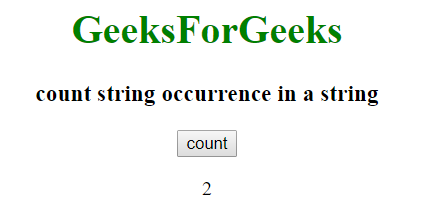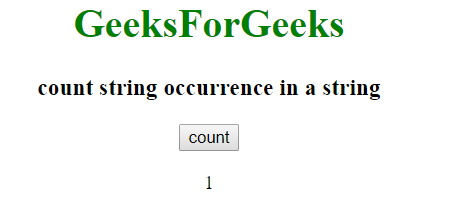# How to count string occurrence in string using JavaScript?

In JavaScript, we can count the string occurrence in a string by counting the number of times the string present in the string. JavaScript provides a function match(), which is used to generate all the occurrences of a string in an array.
By counting the array size which will return the number of times the sub-string present in a string.
Script to find the number of occurrences in a string:

• Example-1: Counting occurrence of Geeks in “GeeksforGeeks” using “match()” function.

 ` ` `<``html``> ` ` `  `<``head``> ` `    ``<``title``> ` `        ``count string occurrence in a string ` `    `` ` ` ` ` `  `<``body` `style``=``"text-align:center;"``> ` ` `  `    ``<``h1` `style``=``"color:green;"``>  ` `        ``GeeksForGeeks  ` `    `` ` `    ``<``h3``> ` `      ``count string occurrence in a string  ` `  `` ` ` `  `    ``<``button` `onClick``=``"gfg()"``> ` `        ``count ` `    `` ` `    ``<``p` `id``=``"rk"``> ` ` `  `    `` ` ` `  `    ``` `    ``<``script``> ` `        ``function gfg() { ` `            ``var r = "Geeks For Geeks "; ` `            ``document.getElementById("rk").innerHTML = ` `              ``(r.match(/Geeks/g)).length; ` ` `  `        ``} ` `    `` ` ` ` ` `  ` `

Output:

• Before clicking on the Button:
•• After clicking on the Button:The ‘g’ in the function specifies global which is used to search for an entire string rather than stop by finding the first occurrence.

• Another Example to count the number of times the string appears in a string.
Example-2: Counting occurrence of for in “GeeksforGeeks” using Loops.

 ` ` `<``html``> ` ` `  `<``head``> ` `    ``<``title``> ` `        ``count string occurrence in a string ` `    `` ` ` ` ` `  `<``body` `style``=``"text-align:center;"``> ` ` `  `    ``<``h1` `style``=``"color:green;"``>  ` `        ``GeeksForGeeks  ` `    `` ` `    ``<``h3``> ` `      ``count string occurrence in a string  ` `  `` ` ` `  `    ``<``button` `onClick``=``"gfg()"``> ` `        ``count ` `    `` ` `    ``<``p` `id``=``"rk"``> ` ` `  `    `` ` ` `  `    ``` `    ``<``script``> ` `        ``function gfg() { ` `            ``var s = "Geeks for Geeks"; ` `            ``var f = "for"; ` `            ``var i = 0, ` `                ``n = 0, ` `                ``j = 0; ` ` `  `            ``while (true) { ` `                ``j = s.indexOf(f, j); ` `                ``if (j >= 0) { ` `                    ``n++; ` `                    ``j++; ` `                ``} else ` `                    ``break; ` `            ``} ` `            ``document.getElementById( ` `              ``"rk").innerHTML = n; ` `        ``} ` `    `` ` ` ` ` `  ` `

Output:

• Before clicking on the Button :
•• After clicking on the Button:• Example-3: Counting occurrence of Geeks in “GeeksforGeeks” using split function.

 ` ` `<``html``> ` ` `  `<``head``> ` `    ``<``title``> ` `        ``count string occurrence in a string ` `    `` ` ` ` ` `  `<``body` `style``=``"text-align:center;"``> ` ` `  `    ``<``h1` `style``=``"color:green;"``>  ` `        ``GeeksForGeeks  ` `    `` ` `    ``<``h3``> ` `      ``count string occurrence in a string  ` `  `` ` ` `  `    ``<``button` `onClick``=``"gfg()"``> ` `        ``count ` `    `` ` `    ``<``p` `id``=``"rk"``> ` ` `  `    `` ` ` `  `    ``` `    ``<``script``> ` `        ``function gfg() { ` `            ``var s = "Geeks for Geeks"; ` `            ``var f = "Geeks"; ` `            ``var r = s.split(f).length - 1; ` `            ``document.getElementById("rk").innerHTML = r; ` `        ``} ` `    `` ` ` ` ` `  ` `

Output:

• Before clicking on the Button:
•• After clicking on the Button:• Example-4:Counting occurrence of Geeks in “GeeksforGeeks” using indexof().

 ` ` `<``html``> ` ` `  `<``head``> ` `    ``<``title``> ` `        ``count string occurrence in a string ` `    `` ` ` ` ` `  `<``body` `style``=``"text-align:center;"``> ` ` `  `    ``<``h1` `style``=``"color:green;"``>  ` `        ``GeeksForGeeks  ` `    `` ` `    ``<``h3``> ` `      ``count string occurrence in a string ` `  `` ` ` `  `    ``<``button` `onClick``=``"gfg()"``> ` `        ``count ` `    `` ` `    ``<``p` `id``=``"rk"``> ` ` `  `    `` ` ` `  `    ``` `    ``<``script``> ` `        ``function gfg() { ` `            ``var s = "Geeks for Geeks"; ` `            ``var f = "Geeks"; ` `            ``var r = s.indexOf(f); ` `            ``var c = 0; ` `            ``while (r != -1) { ` `                ``c++; ` `                ``r = s.indexOf(f, r + 1); ` `            ``} ` `            ``document.getElementById("rk").innerHTML = c; ` `        ``} ` `    `` ` ` ` ` `  ` `

Output:

• Before clicking on the Button :
•• After clicking on the Button:My Personal Notes arrow_drop_upIf you like GeeksforGeeks and would like to contribute, you can also write an article using contribute.geeksforgeeks.org or mail your article to contribute@geeksforgeeks.org. See your article appearing on the GeeksforGeeks main page and help other Geeks.

Please Improve this article if you find anything incorrect by clicking on the "Improve Article" button below.

Article Tags :

Be the First to upvote.

Please write to us at contribute@geeksforgeeks.org to report any issue with the above content.